# Quadrupole mass analyzers: theoretical and practical considerations (Proteomics)

## 1. Introduction

Quadrupole mass analyzers are dynamic mass Alters that play a central role in mass spectrometric proteomic investigations. Quadrupoles are used both alone and in tandem for the isolation (by mass-to-charge) of ions; they may also be used (in RF-only mode) to contain ions during collision-induced dissociation (CID) (Yost and Enke, 1979). Gas-phase ions are generated by a source external to the quadrupole mass analyzer, often at atmospheric pressure. It should be noted that ion production at one atmosphere is an important practical advantage, in that it allows for the easy exchange of ion sources without disturbing the vacuum of the quadrupole region (Cole, 1997). Peptides and proteins are typically introduced into the ion source in the liquid phase either from an HPLC column (for LC/MS), by flow injection analysis (with no HPLC column), or by continuous direct infusion through a fused silica capillary. For heterogeneous samples, a sample cleanup or separation technique such as HPLC or solid-phase extraction is typically incorporated into the method development scheme to allow for the sequential introduction of multiple analytes into the ion source. Simultaneous ionization of multiple analytes, particularly at widely varying concentrations, may result in suppression and prove deleterious in quantitative investigations (Cole, 1997; King etal., 2000; Constantopoulos etal., 1999).

The most common ionization source coupled with quadrupole mass analyzers for proteomics studies is electrospray ionization (ESI), since it is ideal for compounds such as peptides and proteins that can be ionized in solution. The fundamentals of ESI mechanisms have been described elsewhere (Cole, 1997; Kebarle, 2000; Cech and Enke, 2001). A key advantage of ESI is the ability to impose multiple charges on an analyte upon ionization. Recall that ions are separated in mass analyzers as a function of their respective mass-to-charge ratios (m/z) (Throck Watson, 1997). It follows that multiple charging reduces the mass-to-charge ratio of ions, and thereby extends the mass range of the instrument. Atmospheric pressure chemical ionization (APCI) is a highly efficient alternative to ESI for less polar compounds. ESI and APCI are complementary techniques, as reflected in the phase in which charge transfer processes occur (King et al., 2000). Ionization for ESI occurs in the liquid phase, whereas ionization for APCI occurs in the gas phase. Both APCI and ESI are suitable ionization sources for a quadrupole mass analyzer because the ions are generated and transmitted as a continuous beam of ions. The other primary ionization method for proteomics studies is matrix-assisted laser desorption/ionization (MALDI). Since MALDI produces narrow pulses of ions, it is a far better marriage with pulsed mass analyzers (such as time-of-flight mass analyzers; see Article 11, Nano-MALDI and Nano-ESI MS, Volume 5 and Article 14, Sample preparation for MALDI and electrospray, Volume 5) than with the continuous mass analyzers such as quadrupoles. Furthermore, MALDI yields almost exclusively singly charged ions, and thus benefits from the essentially unlimited mass range of the time-of-flight mass analyzer. Quadrupole mass analyzers are also commonly used in mass spectrometers employing electron ionization (EI) and chemical ionization (CI), such as those coupled with gas chromatographs (GC/MS). These ionization techniques do not play a significant role in modern proteomic investigations, however.

The purpose of this communication is to provide the reader with a balanced treatment of practical and theoretical considerations for quadrupole mass analyzers, and to present the advantages of interfacing quadrupoles in tandem.

## 2. Theory and practice of quadrupole mass filters

A quadrupole mass analyzer consists of four electrically isolated hyperbolic or cylindrical rods linked to RF (radio frequency) and DC (direct current) voltages, as described by the schematic in Figure 1 (Pedder, 2001). The combination of RF and DC voltages creates a region of strong focusing and selectivity known as a hyperbolic field. In simplest terms, the ratio of RF/DC allows for the selective transmission of ions of a narrow range of mass-to-charge from the total population of ions introduced from the ionization source.

The idealized hyperbolic field can be described in terms of Cartesian coordinates (x and y directions toward the rods, and z direction along the rods’ axis). Ions of a selected m/z follow a stable trajectory around the center of the field, and are transmitted in the z direction through the device (Dawson and Douglas, 1999). Motion of these “stable ions” in the x and y directions are small in amplitude, as the lowest energy pathway lies toward the center of the hyperbolic field. Ions of other m/z will have unstable trajectories, with increasing displacement in the x and y directions away from the center of the hyperbolic field, and thus strike the quadrupole rods, where they neutralize upon contact. These ions will not transmit efficiently through the quadrupole to the detector. The application of RF and DC voltages creates a region of stability for transmission of ions of a limited m/z range. In this regard, the quadrupole analyzer is operating as a mass filter. A mass spectrum is produced by ramping the RF and DC voltages at a nearly constant ratio. Ions of increasing m/z will sequentially achieve stable trajectories and reach the detector in order of increasing m/z.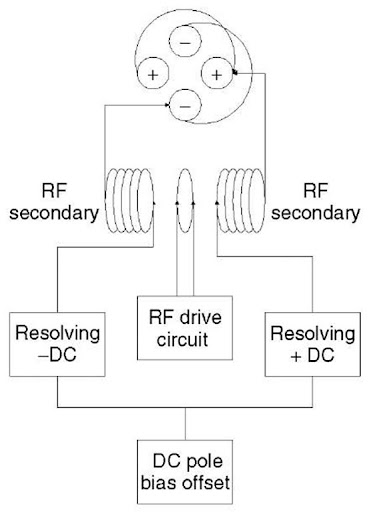Figure 1 The quadrupole mass filter and its power supply

To understand the behavior of ions in the quadrupole, a brief introduction to the mathematics associated with ion motion is worthwhile. For a complete discussion, the reader is referred to the work of March and Hughes (1988).

The motion of ions through a quadrupole is described by a second-order linear differential equation called the Mathieu equation, which can be derived starting from the familiar equation relating force to mass and acceleration, F = ma, yielding the final parameterized form, with the following substitutions for the parameters a and q (Dawson, 1976):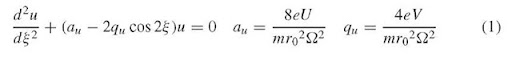The u in the above equations represents position along the coordinate axes (x or y), f is a parameter representing ^t/2, t is time, e is the charge on an electron, U is the applied DC voltage, V is the applied zero-to-peak RF voltage, m is the mass of the ion, r0 is the effective radius between electrodes, and ^ is the applied RF frequency in radians s-1. The parameters a and q are proportional to the DC voltage U and the RF voltage V , respectively.

The analytical solution to this second-order linear differential equation is:

Figure 2 Mathieu stability diagram for an ion of m/z 219 in a quadrupole mass filter with 9.5-mm diameter round rods and an RF frequency of 1.2MHz

which reduces to a similar infinite sum of sine and cosine functions: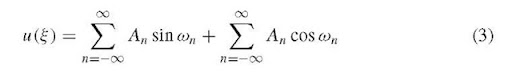The solutions to the Mathieu equation can be presented graphically, as shown in Figure 2 in a so-called stability diagram. Points in (U, V) space (DC, RF voltage space) within the lines lead to stable trajectories for the m/z 219 ion; points outside the lines will lead to an unstable trajectory. The dotted line is called the scan line, and shows the ramp of RF and DC voltage at a nearly constant ratio (the slope of the line). If the line passes just under the apex of the stability region, as shown here, the m/z 219 ions will have a stable trajectory at those voltages, and will be transmitted to the detector.

Figure 3 shows the stability diagram for three different ions, of m/z 28, 69, and 219. Note that ions of lower m/z have stable trajectories at lower RF and DC voltages (i.e., they are stable at points in (U, V) space closer to the origin). The dotted scan line again shows the ramp of RF and DC voltage at a constant ratio (Pedder, 2001). The scan line passes through the apices of the stability regions for all three ions. As the RF and DC voltages ramp up in amplitude, ions of increasing m/z have stable trajectories and are transmitted, as shown in the dotted mass spectrum below the stability diagram. If the scan line has a lower slope (the solid line), it passes through a larger portion of each stability region, resulting in a mass spectrum with lower resolution, as shown as well.

The selection of RF and DC voltages, V and U, the RF frequency, and the inscribed radius r0 between the rods determines the performance of the quadrupole mass filter. Typical values are 4 kV RF(0-p), 700 V DC, 1 MHz RF frequency, and 1 cm radius, and yield a mass-to-charge range of ~3000. As can be noted from equation (4), the Mathieu q parameter is proportional to the RF voltage and inversely proportional to (Constantopoulos et al., 1999; Pedder, 2002)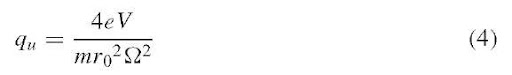the square of the inscribed radius and RF frequency; thus, the mass range of the mass filter can be increased by increasing the RF and DC voltages, or by decreasing the inscribed radius or RF frequency. The mass resolution is a function of the ratio of the RF and DC voltages (the slope of the scan line in Figures 2 and 3); increasing the slope to move the scan line closer to the apex of the stability region (moving from the solid line to the dotted line, for instance) increases the mass resolution, as shown in the spectra in Figure 3(b). Unfortunately, the increase in mass resolution is accompanied by a loss of sensitivity (decrease in transmission of ions through the mass filter). The ultimate resolution that can be achieved is determined not only by how close the scan line approaches the stability region apexes but also by the precision and accuracy of the quadrupole power supplies and the quadrupole rod dimensions.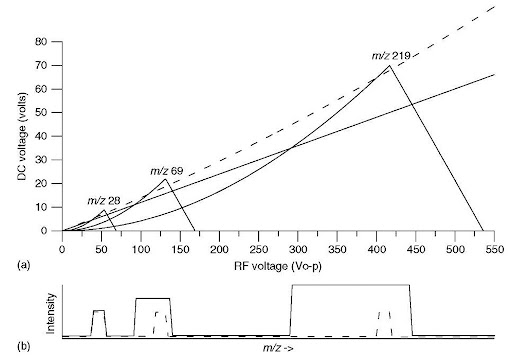Figure 3 (a) Mathieu stability diagram for ions of m/z 28, 69, and 219. Two scan lines are shown, one dotted and a second solid. (b) Simulated mass spectra showing these ions as the RF and DC voltages are scanned along the two scan lines (dotted and solid) shown.

An important operational mode of the quadrupole is achieved when U, the DC potential, is equal to zero (a = 0). This corresponds to a scan line along the x-axis in Figures 2 and 3. This RF-only mode results in transmission of ions of a very wide range of m/z values, and is thus often termed “total ion mode” (Dawson and Douglas, 1999). Note that in this mode, there is a limit to the lowest m/z ion that can achieve a stable trajectory. This can be seen as the right-hand edges of the stability regions in Figure 3. This total ion mode is used for the collision cell in tandem quadrupole mass spectrometers, as discussed below; it is also often employed in quadrupoles used to help transmit ions from the ion source region to the quadrupole or other mass analyzer.

## 3. Tandem mass spectrometry

Most applications of mass spectrometry in proteomics employ mass spectrometry in tandem with one or more other analytical stages (Busch etal., 1998; Niessen, 1999). One of those stages is often a separation stage, typically liquid chromatography or capillary electrophoresis (Cole, 1997), leading to LC/MS and CE/MS, as discussed elsewhere in this volume (see Article 13, Multidimensional liquid chromatography tandem mass spectrometry for biological discovery, Volume 5). Another stage may be a second stage of mass spectrometry (the combination of two or more stages of mass spectrometric analysis in series is termed tandem mass spectrometry (McLafferty 1983; Fetterolf and Yost, 1983) or MS/MS, or even MS”, where n can be >2). Indeed, a common approach in proteomics investigations is combining a stage of separation with tandem mass spectrometry, that is LC/MS/MS and LC/MSn. These tandem (sometimes termed hyphenated) methods offer dramatically improved selectivity and information to solve tough analytical problems, as often encountered in proteomics.

Informing power is a figure of merit that provides a means of quantifying the amount of information available in such an analytical procedure. Fetterolf and Yost (1984) demonstrated the use of informing power to illustrate the advantages of hyphenated methods such as LC/MS/MS over single-stage analytical methods. Note that MS/MS is particularly important in LC/MS (compared to combined gas chromatography/mass spectrometry – GC/MS) for two reasons. First, LC typically provides much poorer chromatographic resolution than GC, meaning that LC separations are often incomplete, with coeluting peaks that MS/MS can help resolve. Second, LC/MS employs ionization methods such as ESI and APCI that provide only molecular-type ions and little or no structural information (GC/MS, in contrast, typically employs EI, which provides significant fragmentation and, therefore, structural information with only a single stage of mass spectrometry). Thus, LC/MS/MS is invaluable in providing structural information for the identification of components eluting from the LC column and for detecting those compound with high selectivity.

MS/MS is readily performed “tandem-in-space” (i.e., with two mass analyzers, typically quadrupole mass filters, in sequence). Note that MS/MS can also be performed “tandem-in-time”, with two or more stages of mass analysis performed sequentially in time in a single ion trap such as a quadrupole ion trap (see Article 9, Quadrupole ion traps and a new era of evolution, Volume 5).

The triple quadrupole mass spectrometer (TQMS, see Figure 4) is the most common MS/MS instrument in use (Yost and Enke, 1979). The operational principles of the triple quadrupole tandem mass spectrometer can be described in a similar fashion to the single quadrupole. Ions from the ion source enter the first quadrupole mass filter (Q1), which selects ions of a selected m/z and transmits them to the second quadrupole. The second quadrupole (Q2) functions as a collision cell in RF-only mode; CID is accomplished by adding a collision gas, typically nitrogen or argon, at a pressure of around 1-10 millitorr (1-10 microbar). The third quadrupole (Q3), another mass filter, provides a means of mass-analyzing the products of CID from the collision cell (Fetterolf and Yost, 1983).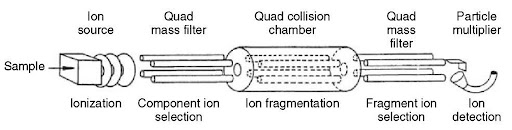Figure 4 Schematic of a triple quadrupole mass spectrometer (TQMS)

Note that the RF-only quadrupole collision cell in triple quadrupole instruments is often replaced with a higher-order multipole (a hexapole or an octopole, with 6 or 8 rods, respectively) (McCloskey, 1990). The use of an RF-only higher-order multipole provides effective transmission of a wider mass range than an RF-only quadrupole. The stability diagram for a hexapole or octopole (as in Figure 2 for the quadrupole) has less well-defined stability boundaries, so the low-mass cut-off characteristic of an RF-only quadrupole is not an issue with higher-order multipole collision cells. A practical advantage that should be noted is that these multipoles require less demanding tuning procedures for the optimization of voltages and frequencies.

A fundamental understanding of the scan modes associated with the TQMS is essential for understanding the MS and MS/MS capabilities of the instrument. These are summarized in Table 1 (McLafferty, 1983; Fetterolf and Yost, 1983; Busch et al., 1998; Dawson and Douglas, 1999).

First, note that the triple quadrupole tandem mass spectrometer can readily be used to perform a single stage of mass spectrometry (MS) by setting two of the three quadrupoles into RF-only (total ion) mode. Either Q1 or Q3 can be used as a mass filter, scanned to obtain a full spectrum, or set to pass a single fixed m/z (or a few fixed m/z values), a mode termed selected ion monitoring (SIM). When Q1 and Q3 are both used as mass analyzers, there are four possible MS/MS scan modes. In the daughter ion scan mode (also called product ion scan), Q3 is scanned to obtain a spectrum of all the daughter ions generated by CID in q2 of the parent or precursor ion mass-selected with Q1. In the parent ion scan mode (also called precursor ion scan), Q1 is scanned to obtain a spectrum of all the parent ions that upon CID in q2 produce the daughter or product ion mass-selected with Q3. In a neutral loss scan, both Q1 and Q3 are scanned at the same rate but with a fixed difference in mass to detect only those ions that undergo a specific neutral loss in q2 such as a loss of mass 18 (H2O). An important MS/MS scan mode for quantitating targeted compounds with maximum sensitivity is the selected reaction monitoring mode (SRM) in which one (or a few) selected parent-ion-to-daughter-ion transitions are monitored by setting Q1 and Q3 to pass specific m/z values. SRM is the MS/MS scan mode analogous to the MS selected ion monitoring scan mode (SIM).

Table 1 MS and MS/MS operational modes of a triple quadrupole mass spectrometer, showing the modes for the two mass filters (Quadrupole 1 and Quadrupole 3) and the quadrupole collision cell (q2)

 Scan mode Q1 q2 Q3 MS – full scana Scan m/z RF-only RF-only MS – selected ion monitoring (SIM)a Fixed m/z RF-only RF-only MS/MS – daughter ion scan Fixed m/z RF-only collision gas Scan m/z MS/MS – parent ion scan Scan m/z RF-only collision gas Fixed m/z MS/MS – neutral loss scan Scan m/z RF-only collision gas Scan m/z b MS/MS – selected reaction monitoring (SRM) Fixed m/z RF-only collision gas Fixed m/z

aNote that the MS scan modes can be performed by mass selection with either Q1 or Q3, with the other one in RF-only (total ion) mode.

bQ3 scanned at a difference in mass corresponding to the mass of the selected neutral.

The relationship between the MS/MS scan modes can be explored by examining the plot of the three-dimensional MS/MS data – intensity (on a logarithmic scale) versus parent ion m/z versus daughter ion m/z – for a single compound, as shown in Figure 5 (Yost and Enke, 1979). Such a three-dimensional data set could be obtained by a series of daughter ion scans of each parent ion formed in the ion source, or by a series of parent ion scans of each daughter ion. It could even be obtained by a series of neutral loss scans for every possible neutral loss (i.e., by interrogating the surface in a series of lines parallel to the front line of the plot). Finally, a series of SRM experiments could measure the intensity of every possible parent ion-daughter ion pair (i.e., every point on the surface).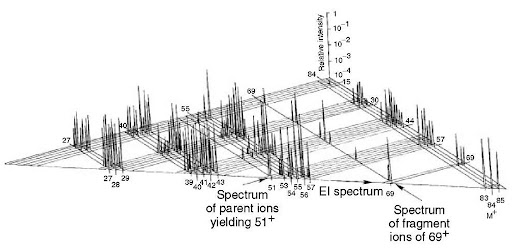Figure 5 Plot of the three-dimensional dataset generated by electron ionization/MS/MS of cyclohexane. A similar plot of 1-propanol could be found in Fetterolf and Yost (1983)

Finally, note that all of these MS and MS/MS scan modes are readily achieved on a TQMS, and indeed, on most tandem-in-space MS/MS instruments. Note that this is not so on tandem-in-time MS/MS instruments, where the selection of a parent ion precedes (temporally, not spatially) the mass selection of the daughter ion. On tandem-in-time MS/MS instruments such as ion traps, parent scans and neutral loss scans are not available scan modes.

## 4. Conclusions

The quadrupole mass analyzer (and the RF-only quadrupole and multipole collision cells) enjoy a central role in the success of mass spectrometric methods in pro-teomics. Development of an understanding of the theoretical and practical aspects of quadrupoles can improve ones ability to utilize single and triple quadrupole mass spectrometers effectively to solve important problems in proteomics.